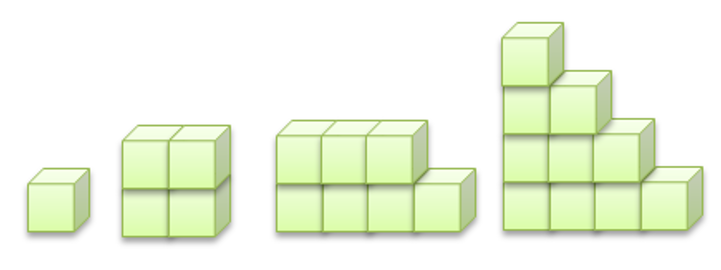Mathematics
Easy

Question

# Smith creates a pattern using the rule “Each layer has 3-blocks”.The number of cubes in the next in Smith’s pattern is

## 10    12    13    16Hint:

## The correct answer is: 13

### We are given a pattern with number of cubes. The rule used to make the pattern is “Each layer has three blocks”.One layer is added every time. With each layer, 3 blocks are added.The number of cubes in the first shape is 1The number of cubes in the second shape is (1 + 3) = 4The number of cubes in the third shape is (4 + 3) = 7The number of cubes in the fourth shape is (7 + 3) = 10So, the number of cubes in fifth shape will be (10 + 3) = 13The number of cubes in the next shape will be 13.

While solving such questions, we should be careful about order of the sequence.

### Related Questions to study#### With Turito Foundation.#### Get an Expert Advice From Turito.